Your No1 source for Latest Entrance Exams, Admission info

 Score 100 percent in English Grammar! Special Course by English Academy Experts - 55 Lessons, 40 Tests for Everyone in CBSE Class 6 to 10 Students - Check out Special Offer englishacademy.successcds.net

Home >> Class 10 >> Science >>

# Magnetic Effects of Electric Current Important Questions 1, 2 and 5 Marks CBSE Class 10 Science Chapter 13

## NCERT Solutions for Class 10 Science Chapter 13 “Magnetic Effects of Electric Current” Important Questions

Here are the Magnetic Effects of Electric Current important questions of 1,2,3 and 5 Marks for CBSE Class 10 Science Chapter 13. The important questions we have compiled will help the students to brush up on their knowledge about the subject. Students can practiceClass 10 Science important questions to understand the subject better and improve their performance in the board exam. The solutions provided here will also give students an idea about how to write the answers. Take Free Online MCQ Test for Class 10

### Magnetic Effects of Electric Current Important Question for CBSE Class 10 Science Chapter 13

Magnetic Effects of Electric Current Important Questions Video

 Magnetic Effects of Electric Current  MCQs 1 Marks Important Questions 2 Marks Important Questions 5 Marks Important Questions

### Magnetic Effects of Electric Current Class 10 Science MCQs (1 Mark)

1. Inside the magnet, the field lines moves
(a) from north to south
(b) from south the north
(c) away from south pole
(d) away from north pole

Answer - a
Explanation: (a) Magnetic field inside the magnet moves from south to north pole.

2. Relative strength of the magnetic field at a point in the space surrounding the magnet is
(a) shown by the length of magnet
(b) thickness of magnet
(c) degree of closeness of the field.
(d) resistance offered by the surroundings

Answer- a
Explanation: (a) The force acting on the pole of another magnet by the crowded magnetic field lines is greater.

3. Which of the following statement is not correct about the magnetic field?
(a) Magnetic field lines form a continuous closed curve.
(b) Magnetic field line do not interest each other.
(c) Direction of tangent at any point on the magnetic field line curve gives the direction of magnetic field at that point.
(d) Outside the magnet, magnetic field lines go from South to North pole of the magnet.

Answer - (d)
Explanation: Outside the magnet, magnetic field line emerges from North-pole and moves towards south-pole.

4. By which instrument, the presence of magnetic field be determined?
(a) Magnetic Needle
(b) Ammeter
(c) Galvanometer
(d) Voltmeter

Answer - a
Explanation: (a) A compass (magnetic needle) can be used to detect presence of magnetic field as it will deflect from its N-S direction to direction if magnetic field at that point.

5. The strength of magnetic field around a current carrying conductor is
(a) inversely proportional to the current but directly proportional to the square of the distance from wire.
(b) directly proportional to the current and inversely proportional to the distance from wire.
(c) directly proportional to the distance and inversely proportional to the current
(d) directly proportional to the current but inversely proportional the square of the distance from wire.

Answer - b
Explanation: Magnetic field strength increases on increasing the current through the wire.
Magnetic field strength decreases as the distance from the wire increases.

6. The strength of each of magnet reduces to half when it cut along its length into the equal parts magnetic field strength of a solenoid. Polarity of solenoid can be determined by
(a) use of compass needle
(b) Right-hand thumb rule
(c) Fleming left-hand rule
(d) either (a) or (b)

Answer - d
Explanation: (d) both (a) and (b) can be used to determine the polarity of the solenoid.

7. The factors on which one magnetic field strength produced by current-carrying solenoids depends are
(a) Magnitude of current
(b) Number of turns
(c) Nature of core material
(d) All of the above

Answer - d
Explanation: (d) Factors shown in (a), (b) and (c).

8. A soft iron bar is introduced inside the current-carrying solenoid. The magnetic field inside the solenoid
(a) Will decrease
(b) Will remains same
(c) Will increase
(d) Will become zero

Answer - c
Explanation: (c) Soft iron inside the current-carrying solenoid act as an electromagnet.

9. Which of the following factors affect the strength of force experienced by current-carrying conduct in a uniform magnetic field?
(a) Magnetic field strength
(b) Magnitude of current in a conductor
(c) Length of the conductor within magnetic field
(d) All of above.

Answer - d
Explanation: (d) All the factors affect the strength of magnetic force.

10. We can induce the current in a coil by
(a) moving the coil in a magnetic field
(b) by changing the magnetic field around it
(c) by changing the orientation of the coil in the magnetic field
(d) All of above

Answer - a
Explanation: (a) The method can be used to induce the potential difference across the ends of a coil and hence induce current.

### Magnetic Effects of Electric Current Class 10 Science 1 Mark Important Questions

Q1. - Give two uses of a magnetic compass.

Answer - It is used to determine the direction of north and south of earth.
It is used to draw the magnetic field lines around a bar magnet.

Q2. - How is the strength of the magnetic field around a wire related to the strength of the electric current flowing in the wire?

Answer - The strength of magnetic field B increases with the increase in the electric current I and vice-versa. That is, Bocl.

Q3. - State the rule used to determine the direction of magnetic field produced around a straight conductor carrying current.

Answer - This rule is used to find the direction of the magnetic field produced by the current-carrying conductor around it.

Statement: If a current-carrying conductor is imagined to be held in the right hand such that the thumb points in the direction of the current, then the curled fingers of the hand indicate the direction of magnetic field.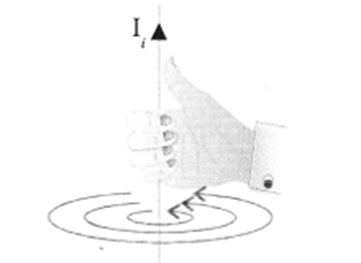Q4 - State the rule to determine the direction of a force experienced by a current-carrying straight conductor placed in a magnetic field which is perpendicular

Answer - Fleming’s left hand rule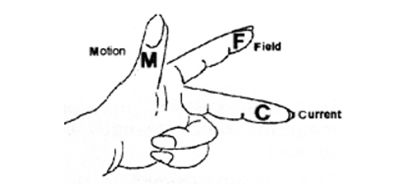Statement: Stretch the left hand such that the thumb, first finger and the central finger are mutually perpendicular to each other.
If the First finger points in the direction of the magnetic Field and the Central finger points in the direction of Current, then the thumb will point in the direction of Motion (or Force)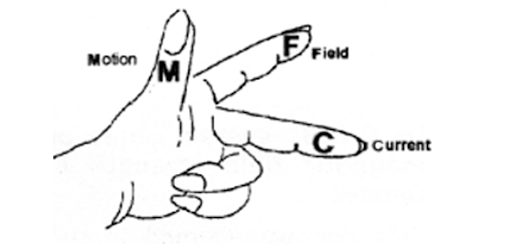Top

### Magnetic Effects of Electric Current Class 10 Science 2 Marks Important Questions

Q1. State the rule to determine the direction of a force experienced by a current-carrying straight conductor placed in a magnetic field which is perpendicular.
Answer - Fleming’s left hand rule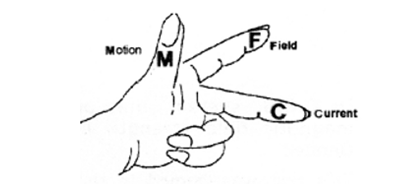Statement: Stretch the left hand such that the thumb, first finger and the central finger are mutually perpendicular to each other.

Q2. Draw a sketch of the pattern of field lines due to a current flowing through a straight conductor.

Answer -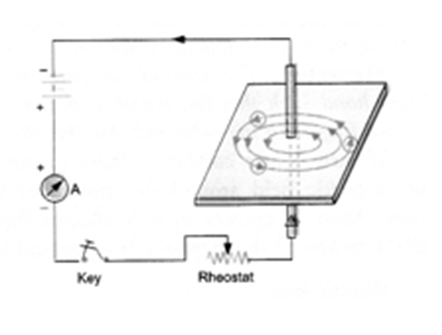If the First finger points in the direction of the magnetic Field and the Central finger points in the direction of Current, then the thumb will point in the direction of Motion (or Force)
Thus, in Fleming’s left hand rule, first finger shows the direction of the magnetic field. The central finger shows the direction of electric current flowing in the conductor.

Thumb shows the direction of force on the conductor or the direction of motion of the conductor.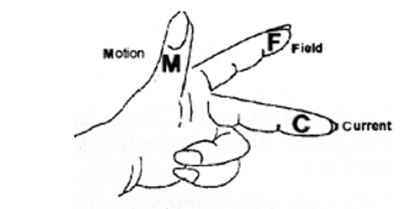Q3. State the rule of determine the direction of current induced in a coil due to its rotation in a magnetic field.

Answer - Fleming stated a rule to determine the direction of induced current in a conductor. This rule is known as
Fleming’s Right-hand rule.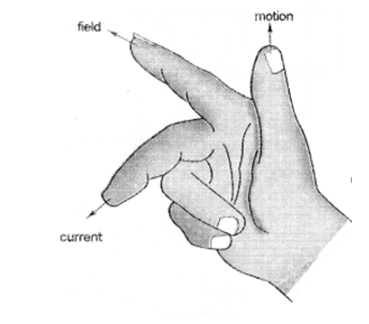Fleming’s Right-hand Rule: “Stretch the right hand such that the first finger, the central finger and the thumb are mutually perpendicular to each other. If the First finger points along the direction of the field (magnetic field) and the Thumb points along the direction of Motion of the conductor, then the direction of induced.

Current is given by the direction of the Central finger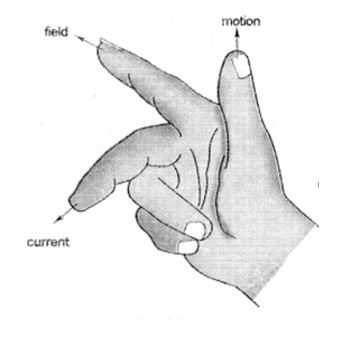Thus, in right-hand rule, the first finger shows the direction of the magnetic field. The central finger
shows the direction of induced current in the conductor. The thumb shows the direction of motion of the conductor in the magnetic field.
Note: Fleming’s Right-hand rule is also known as Dynamo Rule.

Important Videos Links

Top

### Magnetic Effects of Electric Current Class 10 Science 5 Marks Important Questions

Q1. With the help of a labelled circuit diagram describe an activity to illustrate the pattern of the magnetic field lines around a straight current-carrying long conducting wire.
Answer - Describe an activity to plot the magnetic field around a straight conductor carrying current.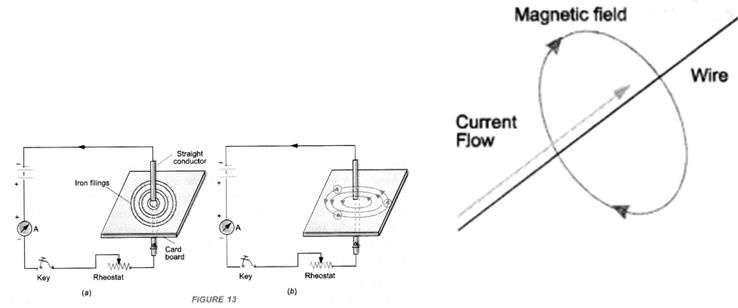1. Take the thick piece of wire consisting of ten turns of insulated copper wire and a cardboard.
2. Pass this thick wire through a hole in the cardboard placed horizontally.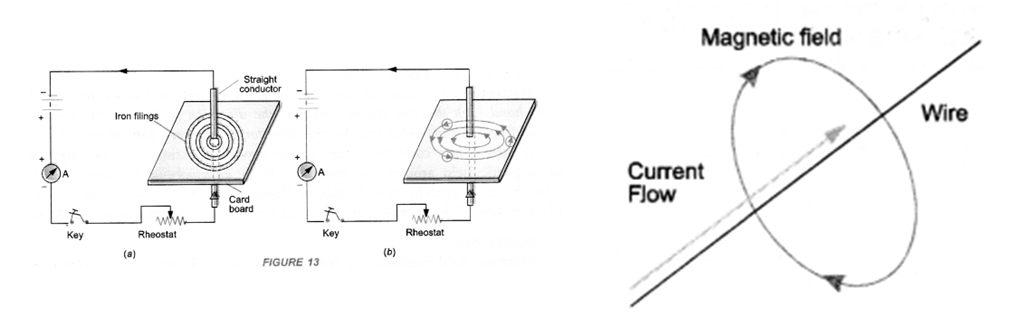Now pass electric current (about 4 A) through the wire by pressing the key and sprinkle some iron filings on the cardboard around the wire.
Tap the cardboard gently.

Observation: We find that the iron filings are arranged in concentric circles around the wire as shown in figure.
If magnetic compass is placed near the current-carrying wire and at different positions, we get concentric circles around the wire as shown in figure These concentric circles around the wire carrying current represent the magnetic field around the wire.

Q2. A coil of insulated wire is connected to a galvanometer. What would be seen if a bar magnet with its south pole towards one face of the coil is
(a) moved quickly towards it?
(b) moved quickly away from it?
(c) placed near its one face?
(d) name the phenomena involved.

Answer - (a) There is deflection in the needle of the galvanometer.
(b) There is deflection in the needle of the galvanometer but in opposite direction.
(c) There is no deflection in the needle of the galvanometer.
(d) Electromagnetic induction

Q3. Explain the meaning of the word “electromagnetic” and “induction” in the term electromagnetic induction.

Answer - “Electromagnetic” means the production of induced current in a closed coil due to the change in magnetic field. “Induction” means the induced current is produced without the actual contact of the closed coil and the moving magnet.

Q4. What are permanent magnet and electromagnets? Give two uses of each.

Answer - Permanent magnet has fixed north and south pole. The strength of the magnetic field of a permanent magnet cannot be changed.
When a soft iron rod is placed inside a current-carrying solenoid, it becomes an electromagnet. The strength of the magnetic field of an electromagnet can be increased or decreased by increasing or decreasing the current flowing in the solenoid. The polarity of electromagnets can be changed by changing the direction of current through the solenoid.

Uses of Permanent Magnet: Permanent magnets are used in loudspeakers and simple electric motors.
Uses of Electromagnet: Electromagnets are used in electric bells, electric horns, television etc.

Q5. Give three differences between an electromagnet and a permanent magnet. (CBSE 2015)
Answer -

 Electromagnet Permanent Magnet 1. It behaves as a magnet as long as electric current passes through the solenoid surrounding it. It is demagnetized when electric current stops passing through the solenoid. Thus, electromagnet is a temporary magnet. 1. It cannot be demagnetized easily. It can be demagnetized either by heating it or throwing it times and again on the floor from certain height. 2. Electromanget gives a strong mangetic field and the strength of the magnetic field produced by the electromagnet can be increased or decreased by increasing or decreasing electric current through the solenoid. 2. Magnetic field of a permanent magnet is weak. The strength of magnetic field of the permanent magnet cannot be changed. 3. The polarity (i.e. North and South poles) of an electromagnet can be reversed by reversing the direction of electric current through the solenoid. 3. The polarity of a permanent magnet cannot be reversed.

Q6. How does the strength of the magnetic field at the centre of a circular coil of a wire depend on
(a) Radius of the coil
(b) Number of turns of the wire of the coil?

Answer - The strength of magnetic field at the centre of the circular coil is
(a) Inversely proportional to the radius of the coil and
(b) Directly proportional to the number of turns of the wire of coil.
Strength of an electromagnet can be increased by increasing the current through solenoid and increasing the number of turns of the solenoid. The polarity of electromagnet is decided by the direction of current flowing in the solenoid.
Uses of electromagnets.
They are used to lift heavy iron pieces. They are fitted on cranes heavy objects of scrap iron.
They are used in many devices like electric bell, electric horn, telephone receiver, electric relay, microphones, radio set, television, loudspeakers etc.

Q7. What is an electromagnet ? What decides its polarity? How it differs from a permanent magnet?

List the three factors and explain how strength of an electromagnet depends on these.

Answer - When a soft iron bar is placed inside a solenoid carrying current, it becomes a magnet as long as current flows through the solenoid. Such a magnet is known as electromagnet.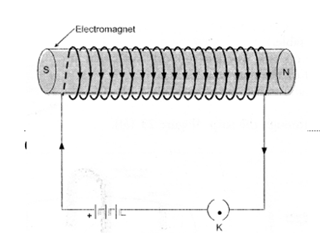Electromagnet Permanent Magnet 1. It behaves as a magnet as long as electric current passes through the solenoid surrounding it. It is demagnetized when electric current stops passing through the solenoid. Thus, electromagnet is a temporary magnet. 1. It cannot be demagnetised easily. It can be demagnetised either by heating it or throwing it times and again on the floor from certain height. 2. Electromanget gives a strong mangetic field and the strength of the magnetic field produced by the electromagnet can be increased or decreased by increasing or decreasing electric current through the solenoid. 2. Magnetic field of a permanent magnet is weak. The strength of magnetic field of the permanent magnet cannot be changed. 3. The polarity (i.e. North and South poles) of an electromagnet can be reversed by reversing the direction of electric current through the solenoid. 3. The polarity of a permanent magnet cannot be reversed.

In fact, the magnetic field inside the solenoid magnetises the soft iron bar placed in it, which acts as an electromagnet.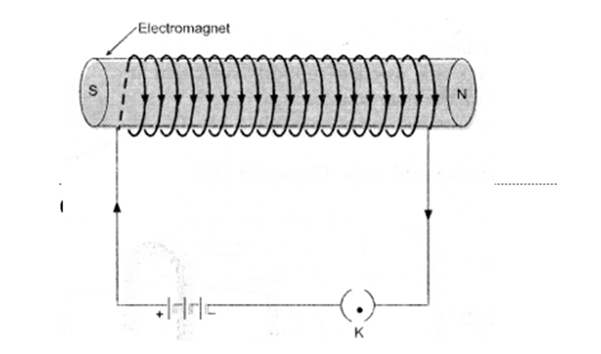Q8. What is the basic difference between AC generator and DC generator?

Answer - In AC generator, slip-ring type commutator is used and in DC generator split-ring type commutator is used.
AC generator converts mechanical energy into electricalenergy in the form of alternating current. DC generator converts mechanical energy into electrical energy in the form of direct current.

Q9. Describe an activity to show how a moving magnet may be used to generate an electric current. State the rule to find the direction of electric current generated in a coil in this manner.

Answer - When a bar magnet moves towards or away from the closed coil, galvanometer shows a deflection. The deflection in the galvanometer indicates that current is flowing through the coil. This electric current is called induced electric current.

It was Michael Faraday, who performed a simple experiment to show that an electric current can be produced in a closed circuit without the use of an electro-chemical cell or battery but by moving a bar magnet towards or away from the closed circuit.

Explanation: When a magnet is moved towards the coil, more and more magnetic field lines pass through the coil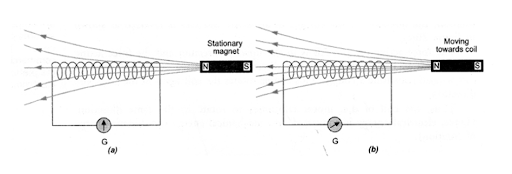Thus, magnetic field around the coil increases. The increase in magnetic field induces potential difference (or voltage) across the ends of the coil. As the coil connected to the galvanometer forms a closed circuit, so induced potential difference makes the current to flow through the circuit. The presence of this current in the circuit is shown by the deflection of the galvanometer.

When a magnet is moved away from the coil, the magnetic field around the coil decreases. The decrease in magnetic field induces potential difference across the ends of the coil. This induced potential difference (or voltage) produces current in the circuit. The presence of the current in the circuit is shown by the deflection of the galvanometer. The current in the circuit is known as induced current.

When the magnet is stationary, the magnetic field around the coil is constant. Therefore, no potential difference is set up across the coil. Hence, no current flows through the circuit.

Direction of Induced Current in a Conductor (Fleming’s Right Hand Rule):

Fleming stated a rule to determine the direction of induced current in a conductor. This rule is known as Fleming’s Right hand rule.

Important Questions Videos Links